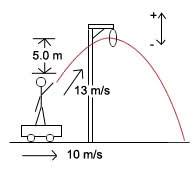# Launching a projectile while

idlackage
Launching a projectile while on something with velocity

## Homework Statement

A man is riding on a cart which is moving at a constant velocity of 10 m/s. He wishes to throw a ball through a stationary hoop 5.0 m above his extended hand in such a manner that the ball will be at the top of its trajectory as it passes through the hoop. He throws the ball with a speed of 13 m/s with respect to himself.

a) What must be the vertical component of the initial velocity of the ball?
b) How many seconds after he releases the ball will it pass through the hoop?
c) At what horizontal distance in front of the hoop must he release the ball?

## Homework Equations

V1 = V2 - a⌂t
⌂d = V1⌂t + 0.5a⌂t2

## The Attempt at a SolutionI pretty much have nothing but that diagram. I have no idea how to even start--I attempted making sense of a triangle calculation somewhere with the givens, but it didn't work out.

Parts b) and c) would be done using one of the 'relevant equations' since I'm guessing I'd obtain the necessary information to plug into them after doing part a).

Thanks for any help.

Last edited:

Homework Helper
How would you go about solving the problem if the cart wasn't moving?

idlackage
How would you go about solving the problem if the cart wasn't moving?

I would start by a component analysis, but since I don't have the angle I can't really do that--which means I'll have to start by finding the angle, but I'm under the impression that all equations I will be using will require components. I know I'm missing some concept but I can't figure it out so it's kind of paradoxical to me right now.

Homework Helper
1. The first (and most important) thing you need are the kinematic equations for a projectile. Do you have those? They should be in your book or you should have instruction on how to derive them. You'll have four: x, vx, y, vy.

2. Starting with y, you know vy at the max height and you know the y distance traveled (from the problem statement). These are two equations with two unknowns that can be solved for t and vy0.

3. You also know v0 from the problem statement. Using the pythagorean theorem you can find vx0.

4. Now that you know t and vx0 you should be able to calculate x.